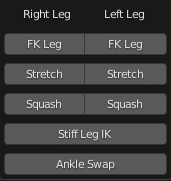# Blender Python Column Align

Ok, so I made a python UI for my rig, and here’s the result.It’s good, but I wanted the buttons to be much closer together. Here’s what mean:The thing is that I don’t know how to do so. Here’s the code:

``````r = b.row(align = True)
r.label(text= "      Right Leg")
r.label(text= "      Left Leg")
r = b.row(align = True)
r.prop(obj, "IK_LEG_RIGHT", text="FK Leg", toggle=True)
r.prop(obj, "IK_LEG_LEFT", text="FK Leg", toggle=True)
r = b.row(align = True)
r.prop(obj, "LRS", text="Stretch", toggle=True)
r.prop(obj, "LLS", text="Stretch", toggle=True)
r = b.row(align = True)
r.prop(obj, "Leg_Squash_R", text="Squash", toggle=True)
r.prop(obj, "Leg_Squash_L", text="Squash", toggle=True)
if rig["IK_LEG_LEFT"] == 0 or rig["IK_LEG_RIGHT"] == 0:
r = b.column(align = True)
r.prop(obj, "Stiff_Leg_IK", text="Stiff Leg IK", toggle=True)
if rig["Stiff_Leg_IK"] == 0:
r = b.column(align = True)
r.prop(obj, "ankle_swap", text="Ankle Swap", toggle=True)
``````

If there are any suggestions. Please let me know.